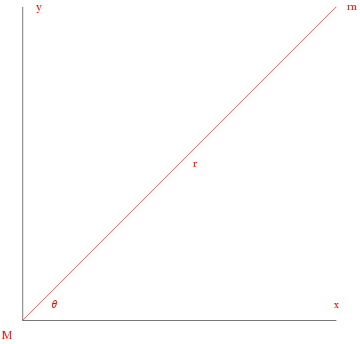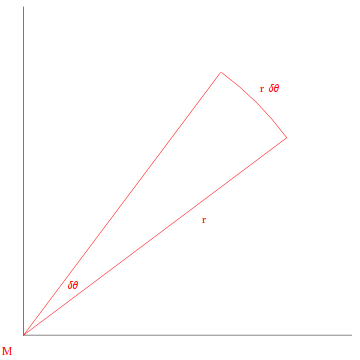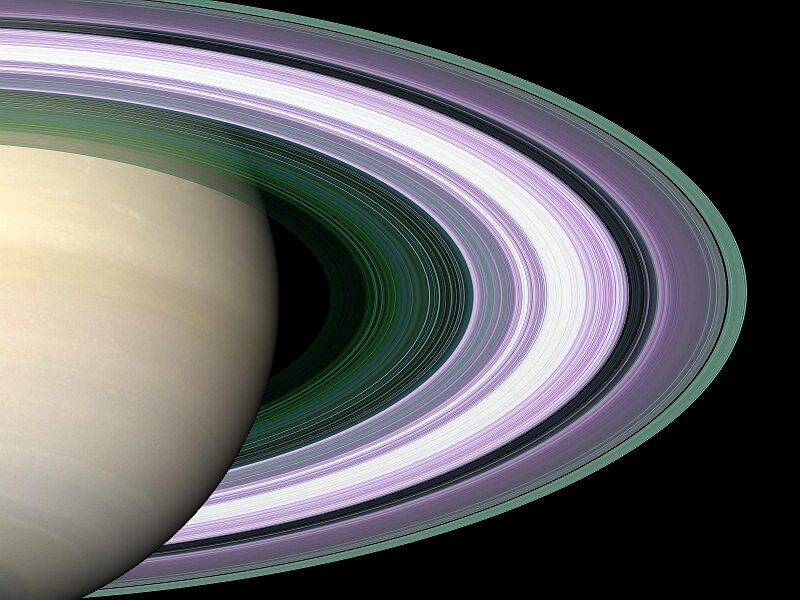# Solar System Dynamics

Using Newton's version of Kepler's third law:
orbital period = 2 * π * (semimajor axis3 / (G * mass))1/2
we can compute the mass of any body with an orbiting satellite using the satellite's orbital parameters. But since we are able to send probe satellites to these bodies, we are able to measure their masses more accurately by measuring their gravitational influence on the paths of our probes and using Newton's Equation:
force due to gravity = G * mass1 * mass2 / distance2
It is enlightening to see how Newton's Equation implies Kepler's laws. Bear with us:
We would like to see how Newton provided a simple and elegant mathematical framework encompassing Kepler's Laws. So we will apply Newton's Law of Gravity to a simple system of a planet and a star, and see if we can derive Kepler's Laws from them.

Let us first assume that the mass m is orbiting in the gravitational field created by the mass M, and that m is spherical and far enough from M that it can be considered a point mass. Considering the enormous distances between them relative to their sizes, this is not a problem.

Newton's law of gravity is written

F = - G m M / r2
or, using his Second law (force = mass * acceleration), as
m a = - G m M / r2
From this we see immediately that Newton's law of gravity DOES NOT DEPEND ON m .

In Einstein's General Relativity, this is a result of the Equivalence Principle, which states that Gravity acts the same on all masses regardless of their composition: the mass's acceleration does not depend on the mass itself.

Rearranging, we then have

(G M) / r2 + a = 0
But there is another piece of information that is hidden in Newton's law of gravity: THE FORCE ACTS ON A LINE BETWEEN THE TWO MASSES.

Since two points define a plane, this is a planar problem, meaning that we have to think in terms of x and y directions. We lose no generality by choosing a coordinate system so that M is at the origin and m is at {x[t], y[t]}. Since acceleration is the rate of change of the rate of change of the position with time, we write a as

{x''[t], y''[t]}
(where each prime indicates "rate of change") and multiply the (G M)/r2 term by the direction {x[t], y[t]}/r, where r is √(x2+y2) (using the Pythagorean theorem). Newton's law then becomes a pair of equations:
G M x[t] / √(x[t]2 + y[t]2)3 + x''[t]=0,
G M y[t] / √(x[t]2 + y[t]2]3 + y''[t]=0
This is a miserable pair of equations to solve, but if we express them in terms of r and the angle θ between the line connecting m and M, and the x axis:we can attack them more simply.

First recall that x/r = cos θ and y/r = sin θ. Making these substitutions into our pair of equations and using the calculus that Newton invented for the occasion gives us two equivalent equations that are much more tractable:

G M / r[t]2 - r[t] θ'[t]2 + r''[t]=0,
r[t]2 θ'[t] = J
In the second equation, J is a constant called the angular momentum. It measures both the rate of rotation and the bulk of the rotating object. Since the area of a triangle is one half the base times the height, r[t]2 θ'[t] is twice the rate at which area is swept out by the path r[t]:So we have just found Kepler's second law as a result of Newton's law of gravity: the area swept out in any given time is a constant.

With some significant but perfectly legal manipulations (involving more of Newton's calculus), we can make the substitution u=1/r, trade t dependence for θ dependence, and change the other equation to

u''[θ] + u[θ] = G M / J2
This has the solution (after we substitute 1/r for u)
r = ((1 + ε) r0) / (1 + ε Cos[θ])
Here, we have traded the two physical constants describing the motion (M and J) for two geometrical constants which describe the orbit: (G M)/J2 is 1/(r0(1+ε)), where r0 is the perihelion and ε is the eccentricity of the ellipse describing the orbit.

As expected, M is at one focus: the origin. So we have found Kepler's first law as well.

Since the rate at which area is swept out is J/2, the time it takes to complete one orbit (the period) is the area of the orbit (π a b) divided by J/2. Now the length of the semimajor axis (a) is (r+r[π])/2, or

(r0 + r0 (1 + ε) / (1 - ε)) / 2 = r0 / (1 - ε)
and the length of the semiminor axis (b) is a √(1 - ε2). The period of the orbit is then
(2 π a b) / J = (2 π r02 √(1 - ε2)) / (J (1 - ε)2)
Since J is √(G M r0 (1 + ε)), the period is
2 π √(1 / (G M)) (r0 / (1 - ε))(3/2) = 2 π √(a3 / (G M))
and we have just found Kepler's third law: the orbital period is proportional to the square root of the cube of the semimajor axis.

There is something else pretty special here. It turns out (Bertrand proved it) that the inverse square force law is one of only TWO force laws which allow closed orbits. And the inverse square law is a direct consequence of the fact that we live in 3 dimensions.

Now this was all for one planet and one star, but Newton's laws are powerful enough for us to use them for more complex systems; just not in this text.

And it turns out that we have actually accomplished more than we thought we did. All this time you thought (admit it!) that M was the mass of the star. But since the gravitational force is felt by both the star and the planet, the planet will cause the star to wobble, so it cannot be fixed at any point. (This is how we most often detect extrasolar planets.)

What we have actually done is find both the motion of the planet and of the star: each orbits in an ellipse around their common center of mass, and M is the total mass (star plus planet). The origin is the location of the center of mass of the star-planet system. Since the star is so much more massive than the planet, the center of mass is very close to the center of the star, so we observe the elliptical orbit of the star as the wobble we spoke of above.

What about our treatment of the planet as a point mass? Gauss' law tells us that the gravitational force due to a spherically symmetric mass is equivalent to the force due to a point particle of the same mass, located at the center. Therefore we will usually treat planets (and stars) as if all their mass was located at a point at their center.

angular momentum = 2 * π * mass * rotation rate * orbital radius2)
and their central pressures. The last we can estimate from dimensional considerations, using the formula
pressure = G * mass2 / radius4
Note that this value of the Earth's central pressure is 14.23 million times the atmospheric pressure on the surface.

BodyM(kg)M(Earth)Densityg(Earth)L (kgm2/s) L(Earth)Central Pres.Central Pres.
(g/cm3)(Pa)(Earth)
Sun1.989 * 10303.33 * 1051.411281.424 * 1056 5.349 * 10151.128 * 1015784.1
Mercury3.302 * 10230.055285.4290.37789.149 * 1038 0.034372.054 * 10110.1427
Venus4.868 * 10240.8155.2440.90531.846 * 1040 0.69341.179 * 10120.8195
Earth5.974 * 102415.49612.662 * 1040 11.439 * 10121
The Moon7.348 * 10220.01233.3650.16642.884 * 1034 1.083 * 10-63.986 * 10100.0277
Mars6.418 * 10230.10743.9090.37883.531 * 1039 0.13262.064 * 10110.1435
Phobos1.063 * 10161.779 * 10-90.96233.792 * 10-42.132 * 1026 8.009 * 10-152.07 * 1051.438 * 10-7
Deimos2.38 * 10153.984 * 10-101.252.742 * 10-47.545 * 1025 2.835 * 10-151.082 * 1057.52 * 10-8
Ceres8.7 * 10201.456 * 10-41.9630.02656.453 * 1036 2.424 * 10-41.009 * 1097.013 * 10-4
Eros6.69 * 10151.12 * 10-91.23.765 * 10-43.6 * 1031 1.353 * 10-92.039 * 1051.417 * 10-7
Gaspra10161.674 * 10-93.9879.587 * 10-46.609 * 1031 2.483 * 10-91.323 * 1069.192 * 10-7
Ida10171.674 * 10-82.8751.661 * 10-37.536 * 1032 2.831 * 10-83.969 * 1062.758 * 10-6
Mathilde1.033 * 10171.729 * 10-80.70896.599 * 10-47.083 * 1032 2.661 * 10-86.266 * 1054.355 * 10-7
Vesta3 * 10205.022 * 10-53.8490.02912.055 * 1036 7.719 * 10-51.218 * 1098.464 * 10-4
Jupiter1.898 * 1027317.81.242.531.932 * 1043 725.89.209 * 10126.4
Io8.932 * 10220.014953.5310.18346.538 * 1035 2.456 * 10-54.841 * 10100.03364
Europa4.8 * 10220.0080352.9890.13354.426 * 1035 1.663 * 10-52.563 * 10100.01781
Ganymede1.482 * 10230.024811.9360.14551.725 * 1036 6.479 * 10-53.044 * 10100.02116
Callisto1.076 * 10230.018011.8510.12691.662 * 1036 6.245 * 10-52.317 * 10100.0161
Saturn5.685 * 102695.170.621.0667.837 * 1042 294.41.635 * 10121.136
Mimas3.75 * 10196.278 * 10-61.1890.0066489.96 * 1031 3.742 * 10-96.359 * 1074.419 * 10-5
Enceladus7. * 10191.172 * 10-51.1090.0078142.105 * 1032 7.907 * 10-98.785 * 1076.105 * 10-5
Tethys6.27 * 10201.05 * 10-41.0050.01522.097 * 1033 7.879 * 10-83.325 * 1082.311 * 10-4
Dione1.1 * 10211.841 * 10-41.4950.023894.163 * 1033 1.564 * 10-78.21 * 1085.706 * 10-4
Rhea2.31 * 10213.867 * 10-41.2370.026951.033 * 1034 3.881 * 10-71.045 * 1097.263 * 10-4
Titan1.346 * 10230.022521.8810.13829.161 * 1035 3.442 * 10-52.748 * 10100.0191
Hyperion8 * 10171.339 * 10-70.27296.912 * 10-45.993 * 1030 2.251 * 10-106.875 * 1054.778 * 10-7
Iaepetus1.6 * 10212.678 * 10-41.0320.021141.86 * 1034 6.989 * 10-76.428 * 1084.467 * 10-4
Uranus8.685 * 102514.541.2370.90321.696 * 1042 63.731.174 * 10120.8157
Miranda6.6 * 10191.105 * 10-51.1990.008075.728 * 1031 2.152 * 10-99.371 * 1076.512 * 10-5
Ariel1.35 * 10212.26 * 10-41.660.027421.42 * 1033 5.336 * 10-81.082 * 1097.52 * 10-4
Titania3.53 * 10215.909 * 10-41.7160.038635.613 * 1033 2.109 * 10-72.147 * 1090.001492
Neptune1.024 * 102617.151.611.1382.505 * 1042 94.091.862 * 10121.294
Triton2.14 * 10220.0035822.0670.07973-3.333 * 1034 -1.252 * 10-69.146 * 1090.006356
Several densities of interest:

• liquid water at 4 C - 1 g / cm3
• gaseous H2 at 0 C and 1 atm - .00009 g / cm3
• metallic H - 1.3 to 1.6 g / cm3
• silicate minerals - 3 to 4 g / cm3
• ferric/ferrous minerals - 7 to 8 g / cm3
Two other quantities worth noting are the orbital velocity (obtained from equating the gravitational acceleration to the acceleration v2/r required to keep a body moving in a circular orbit):
orbital velocity = (G * mass / distance)1/2
and the escape velocity (obtained from equating the kinetic energy mv2/2 to the gravitational energy Gm/r):
escape velocity = (2 * G * mass / distance)1/2
In both of these equations, the mass is the mass of the body we are orbiting or escaping from; the results are independent of the mass of our spacecraft. The distance in each equation is the distance from the center of the body (its radius in the case of the escape velocity).

Portfolio Exercise: Use the relation between the orbital period, the semimajor axis length and the total mass, to compute the masses of the Sun and those planets with moons.
Recall from our derivation of Kepler's Laws from Newton's that the mass in this relation is the combined mass of the parent and the satellite. For this reason, you will get the best results if you choose the satellite with the lowest mass (ie., use Mercury's orbital parameters to compute the mass of the Sun, Deimos' to compute Mars' mass, etc.).
Compare these values to the measured values quoted above: in terms of a percentage, how far off are your values from those in the table above?

For each body in the table above, compute the orbital velocity at a distance of 400 km from the surface, and the escape velocity from the surface. Compute each value in both km/hr and in multiples of the Earth's value.

### Gravity and Tides

Using the masses above, it is interesting to compare the strengths of the gravitational forces among some of these bodies. This table gives the gravitational force between some of the planets and their moons, expressed as a fraction of the gravitational force between the Sun and that planet:

PlanetBodyFgrav (Sun)
EarthThe Moon0.005594
JupiterIo0.1528
JupiterEuropa0.03248
JupiterGanymede0.03943
JupiterCallisto0.009244
SaturnJupiter0.004623
SaturnTethys0.00739
SaturnDione0.007904
SaturnRhea0.008511
SaturnTitan0.09224
UranusMiranda0.01622
UranusAriel0.1534
UranusTitania0.07685
NeptuneTriton1.73

We can see that many of the moons have a significant perturbative influence on the orbital motion of their planets.

It is also interesting to compare the tidal accelerations caused by some of these bodies on others. We will define the tidal acceleration as the difference between the gravitational acceleration on the near side of the body and that at its center:

accelerationtidal = G * mass1 * (1 / (distance - radius2)2 - 1 / distance2)
where the distance is the distance between the bodies, and the subscripts refer to their labels below. These values have been expressed as fractions of the tidal acceleration of the Moon on the Earth (1.286 * 10-6 m/s2), and as fractions of the body's own surface gravity.
Here is a time lapse movie of tidal basin filling (17.83 Mb), and one of a tidal bore (6.87 Mb) (the turbulence downstream of a narrow opening).
In all cases, the length of the orbital semimajor axis has been used to compute the distance:

Body 1Body 2atidal (Moon on Earth)atidal / g
SunMercury2.9559.008 * 10-7
SunVenus1.1231.429 * 10-7
SunEarth0.44815.161 * 10-8
SunThe Moon0.12188.43 * 10-8
EarthThe Moon21.71.502 * 10-5
The MoonEarth11.152 * 10-7
MarsPhobos12740.387
MarsDeimos45.220.01899
JupiterIo54760.003438
JupiterEuropa11670.001007
JupiterGanymede484.53.837 * 10-4
JupiterCallisto80.967.349 * 10-5
IoJupiter13.336.069 * 10-7
IoEuropa1.0819.327 * 10-7
IoGanymede0.10298.146 * 10-8
EuropaJupiter1.5937.252 * 10-8
EuropaIo0.67684.25 * 10-7
EuropaGanymede0.23771.882 * 10-7
GanymedeJupiter1.1355.168 * 10-8
GanymedeIo0.11787.395 * 10-8
GanymedeEuropa0.43433.748 * 10-7
CallistoJupiter0.14446.574 * 10-9
SaturnMimas20670.03581
SaturnTethys13960.01058
SaturnDione701.90.003384
SaturnRhea351.60.001503
SaturnTitan95.27.935 * 10-5
SaturnHyperion1.8373.062 * 10-4
SaturnIaepetus1.0695.825 * 10-6
TethysSaturn0.24782.677 * 10-8
DioneSaturn0.192.053 * 10-8
DioneTethys0.12299.31 * 10-7
RheaSaturn0.13521.461 * 10-8
TitanSaturn0.56736.13 * 10-8
TitanHyperion0.081131.352 * 10-5
UranusMiranda11090.01583
UranusAriel8580.003604
UranusTitania97.812.917 * 10-4
MirandaUranus0.12751.626 * 10-8
ArielUranus0.739.309 * 10-8
ArielMiranda0.16632.374 * 10-6
TitaniaUranus0.14091.797 * 10-8
NeptuneTriton368.95.33 * 10-4
TritonNeptune1.5651.585 * 10-7

All of the moons in this table are locked in synchronous orbits: their rotational period is equal to their orbital period. This is due to tidal deformations (bulges toward the planet) which hold the same face toward the planet at all times. Mercury is also in a sychronous orbit, but in a more complicated fashion because of its higher eccentricity: it rotates 3 times for every 2 orbits around the Sun.

In addition, it is interesting to compare the tidal accelerations to that of Europa on Io, which is thought to account for much of Io's internal heating, and subsequent active volcanism. Note that values in the final column are all less than 1. If the tidal acceleration were equal to or greater than the surface gravity, the body would be gravitationally unstable and would break up. This happens to bodies lying withing the Roche Limit.

Saturn's Rings lie within Saturn's Roche Limit. (source)In this image, green hues indicate particle sizes less than 5 cm, while blue indicates less than 1 cm. Purple regions had pieces larger than 5 cm (up to several meters), and white indicates an area so dense that it was opaque to the radio waves used to probe the particle sizes.